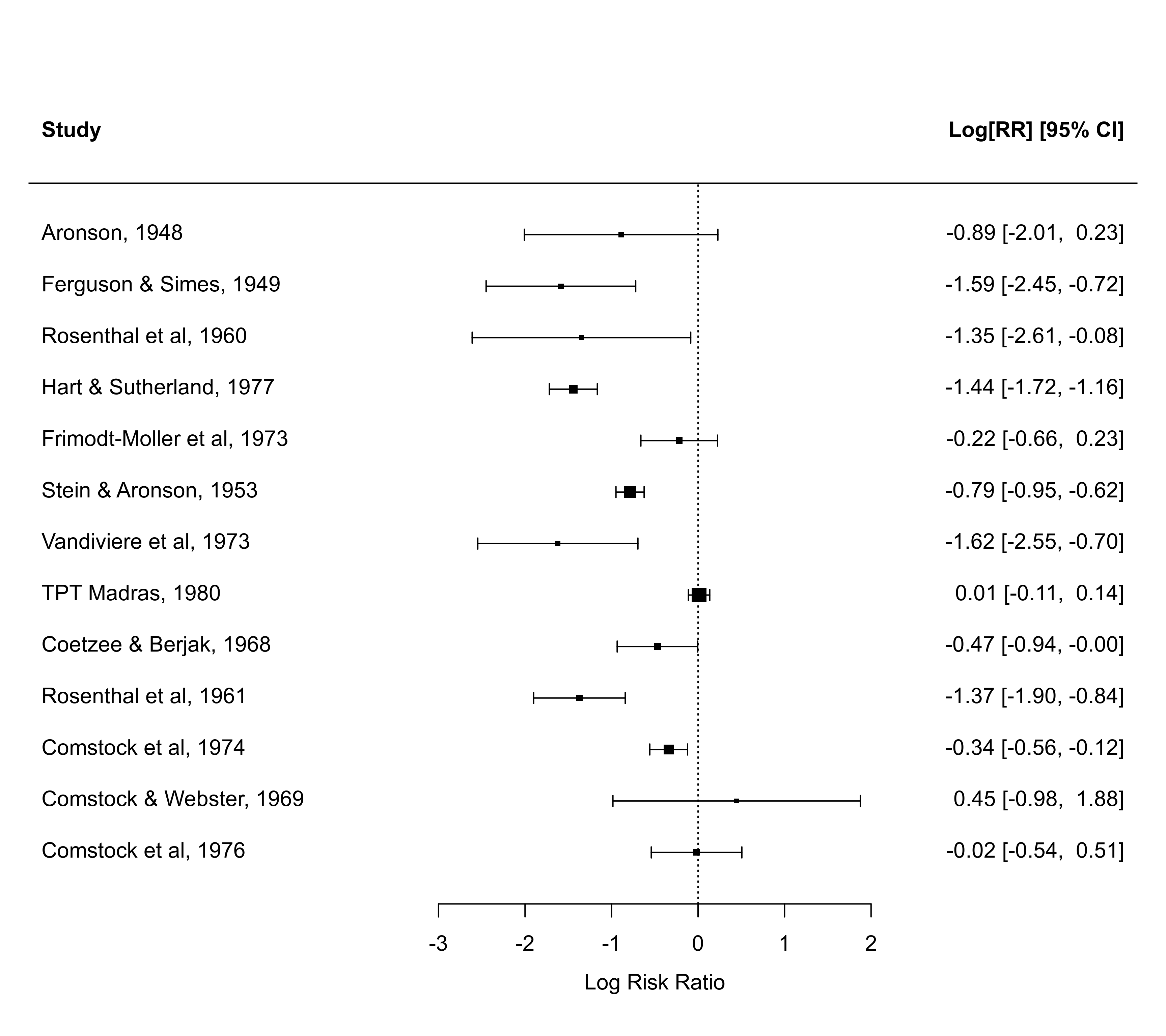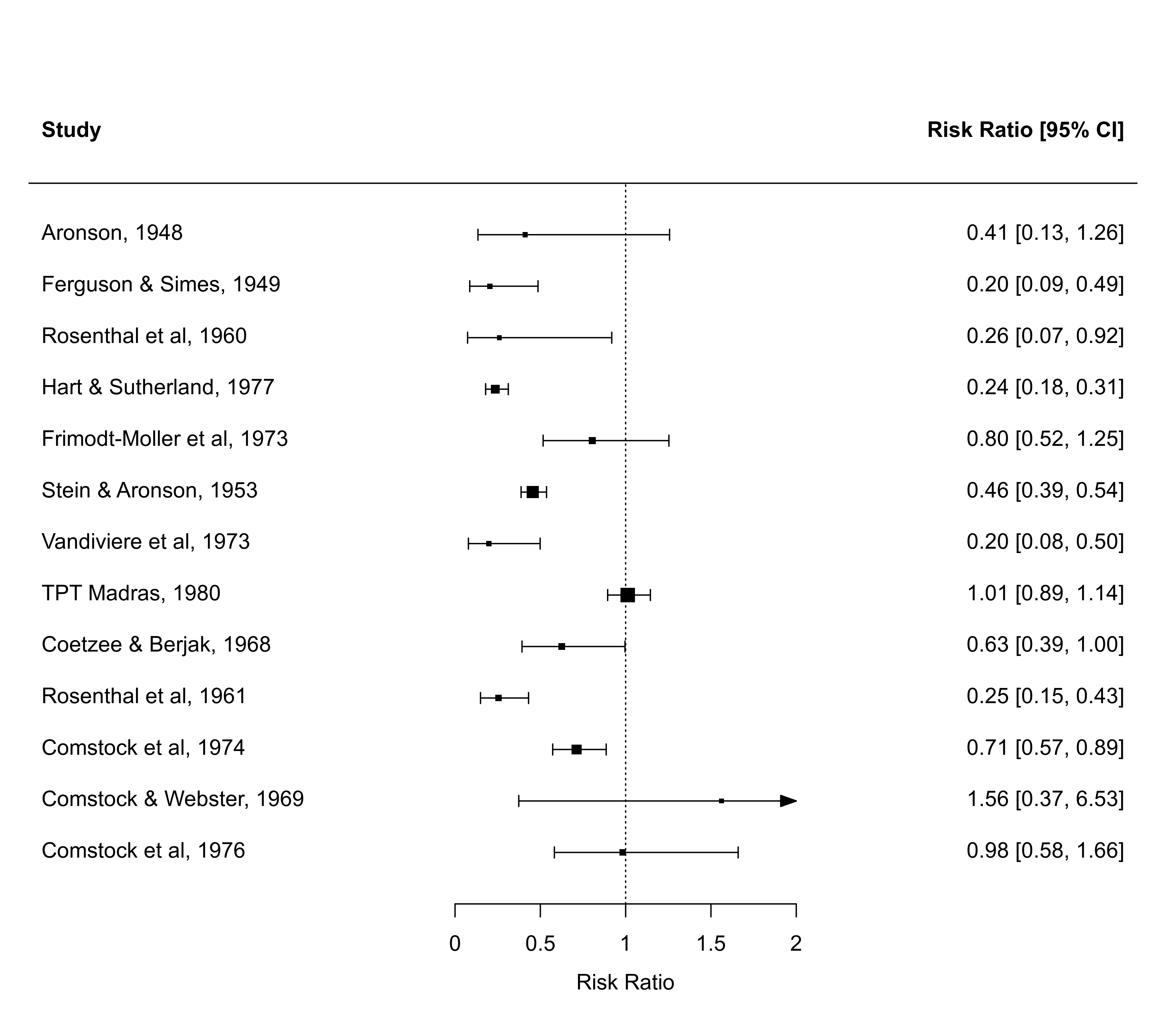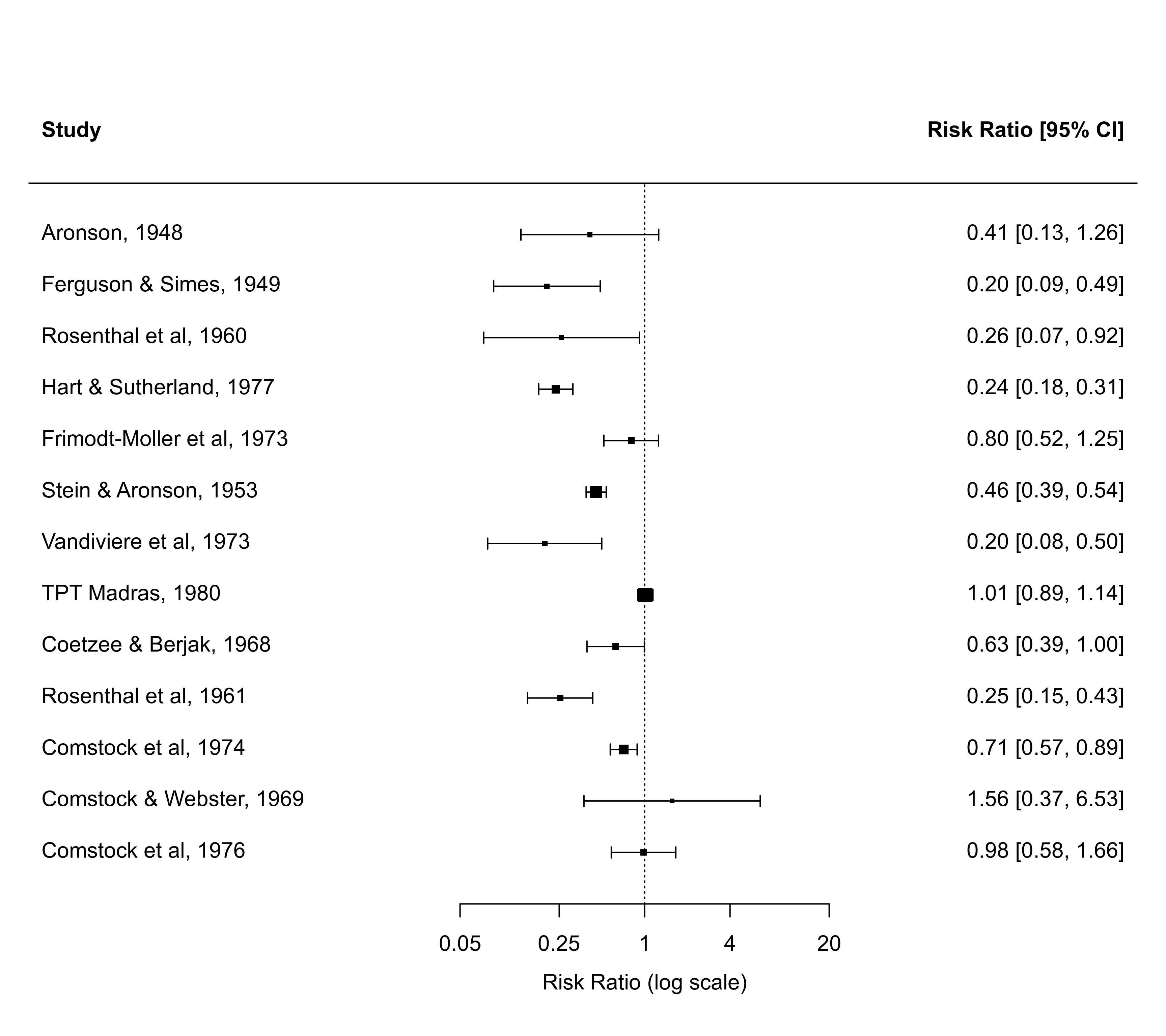Function to create forest plots for a given set of data.

# S3 method for default
forest(x, vi, sei, ci.lb, ci.ub,
xlim, alim, olim, ylim, at, steps=5,
level=95, refline=0, digits=2L, width,
xlab, slab, ilab, ilab.xpos, ilab.pos,
order, subset, transf, atransf, targs, rows,
efac=1, pch, psize, plim=c(0.5,1.5), col,
lty, fonts, cex, cex.lab, cex.axis, ...)

## Arguments

x

vector of length $$k$$ with the observed effect sizes or outcomes.

vi

vector of length $$k$$ with the corresponding sampling variances.

sei

vector of length $$k$$ with the corresponding standard errors (note: only one of the two, vi or sei, needs to be specified).

ci.lb

vector of length $$k$$ with the corresponding lower confidence interval bounds. Not needed if vi or sei is specified. See ‘Details’.

ci.ub

vector of length $$k$$ with the corresponding upper confidence interval bounds. Not needed if vi or sei is specified. See ‘Details’.

annotate

logical to specify whether annotations should be added to the plot (the default is TRUE).

showweights

logical to specify whether the annotations should also include the inverse variance weights (the default is FALSE).

logical to specify whether column headings should be added to the plot (the default is FALSE). Can also be a character vector to specify the left and right headings (or only the left one).

xlim

horizontal limits of the plot region. If unspecified, the function tries to set the horizontal plot limits to some sensible values.

alim

the x-axis limits. If unspecified, the function tries to set the x-axis limits to some sensible values.

olim

optional argument to specify observation/outcome limits. If unspecified, no limits are used.

ylim

the y-axis limits of the plot. If unspecified, the function tries to set the y-axis limits to some sensible values.

at

position of the x-axis tick marks and corresponding labels. If unspecified, the function tries to set the tick mark positions/labels to some sensible values.

steps

the number of tick marks for the x-axis (the default is 5). Ignored when the positions are specified via the at argument.

level

numeric value between 0 and 100 to specify the confidence interval level (the default is 95).

refline

numeric value to specify the location of the vertical ‘reference’ line (the default is 0). The line can be suppressed by setting this argument to NA.

digits

integer to specify the number of decimal places to which the tick mark labels of the x-axis and the annotations should be rounded (the default is 2L). Can also be a vector of two integers, the first to specify the number of decimal places for the annotations, the second for the x-axis labels. When specifying an integer (e.g., 2L), trailing zeros after the decimal mark are dropped for the x-axis labels. When specifying a numeric value (e.g., 2), trailing zeros are retained.

width

optional integer to manually adjust the width of the columns for the annotations (either a single integer or a vector of the same length as the number of annotation columns).

xlab

title for the x-axis. If unspecified, the function tries to set an appropriate axis title.

slab

optional vector with labels for the $$k$$ studies. If unspecified, simple labels are created within the function. To suppress labels, set this argument to NA.

ilab

optional vector, matrix, or data frame providing additional information about the studies that should be added to the plot.

ilab.xpos

numeric vector to specify the horizontal position(s) of the variable(s) given via ilab (must be specified if ilab is specified).

ilab.pos

integer(s) (either 1, 2, 3, or 4) to specify the alignment of the vector(s) given via ilab (2 means right, 4 mean left aligned). If unspecified, the default is to center the labels.

order

optional character string to specify how the studies should be ordered. Can also be a variable based on which the studies will be ordered. See ‘Details’.

subset

optional (logical or numeric) vector to specify the subset of studies that should be included in the plot.

transf

optional argument to specify a function to transform the observed outcomes and corresponding confidence interval bounds (e.g., transf=exp; see also transf). If unspecified, no transformation is used.

atransf

optional argument to specify a function to transform the x-axis labels and annotations (e.g., atransf=exp; see also transf). If unspecified, no transformation is used.

targs

optional arguments needed by the function specified via transf or atransf.

rows

optional vector to specify the rows (or more generally, the horizontal positions) for plotting the outcomes. Can also be a single value to specify the row (horizontal position) of the first outcome (the remaining outcomes are then plotted below this starting row). If unspecified, the function sets this value automatically.

efac

vertical expansion factor for confidence interval limits and arrows. The default value of 1 should usually work okay. Can also be a vector of two numbers, the first for CI limits, the second for arrows.

pch

plotting symbol to use for the observed outcomes. By default, a filled square is used. See points for other options. Can also be a vector of values.

psize

optional numeric value to specify the point sizes for the observed outcomes. If unspecified, the point sizes are a function of the precision of the estimates. Can also be a vector of values.

plim

numeric vector of length 2 to scale the point sizes (ignored when psize is specified). See ‘Details’.

col

optional character string to specify the color to use for plotting the observed outcomes ("black" is used by default if not specified). Can also be a vector.

lty

optional character string to specify the line type for the confidence intervals. If unspecified, the function sets this to "solid" by default.

fonts

optional character string to specify the font to use for the study labels, annotations, and the extra information (if specified via ilab). If unspecified, the default font is used.

cex

optional character and symbol expansion factor. If unspecified, the function tries to set this to a sensible value.

cex.lab

optional expansion factor for the x-axis title. If unspecified, the function tries to set this to a sensible value.

cex.axis

optional expansion factor for the x-axis labels. If unspecified, the function tries to set this to a sensible value.

...

other arguments.

## Details

The plot shows the observed effect sizes or outcomes with corresponding confidence intervals. To use the function, one should specify the observed outcomes (via the x argument) together with the corresponding sampling variances (via the vi argument) or with the corresponding standard errors (via the sei argument). Alternatively, one can specify the observed outcomes together with the corresponding confidence interval bounds (via the ci.lb and ci.ub arguments).

With the transf argument, the observed outcomes and corresponding confidence interval bounds can be transformed with some suitable function. For example, when plotting log odds ratios, then one could use transf=exp to obtain a forest plot showing the odds ratios. Alternatively, one can use the atransf argument to transform the x-axis labels and annotations (e.g., atransf=exp). See also transf for some other useful transformation functions in the context of a meta-analysis. The examples below illustrate the use of these arguments.

By default, the studies are ordered from top to bottom (i.e., the first study in the dataset will be placed in row $$k$$, the second study in row $$k-1$$, and so on, until the last study, which is placed in the first row). The studies can be reordered with the order argument:

• order="obs": the studies are ordered by the observed outcomes,

• order="prec": the studies are ordered by their sampling variances.

Alternatively, it is also possible to set order equal to a variable based on which the studies will be ordered (see ‘Examples’).

Additional columns with information about the studies can be added to the plot via the ilab argument. This can either be a single variable or an entire matrix / data frame (with as many rows as there are studies in the forest plot). The ilab.xpos argument must then also be specified to indicate the horizontal position of the variables specified via ilab.

Summary estimates can be added to the plot with the addpoly function. See the documentation for that function for examples.

By default (i.e., when psize is not specified), the point sizes are a function of the precision (i.e., inverse standard errors) of the outcomes. This way, more precise estimates are visually more prominent in the plot. By making the point sizes a function of the inverse standard errors of the estimates, their areas are proportional to the inverse sampling variances, which corresponds to the weights they would receive in an equal-effects model. However, the point sizes are rescaled so that the smallest point size is plim and the largest point size is plim. As a result, their relative sizes (i.e., areas) no longer exactly correspond to their relative weights in such a model. If exactly relative point sizes are desired, one can set plim to NA, in which case the points are rescaled so that the smallest point size corresponds to plim and all other points are scaled accordingly. As a result, the largest point may be very large. Alternatively, one can set plim to NA, in which case the points are rescaled so that the largest point size corresponds to plim and all other points are scaled accordingly. As a result, the smallest point may be very small and essentially indistinguishable from the confidence interval line. To avoid the latter, one can also set plim, which enforces a minimal point size.

## Note

The function tries to set some sensible values for the optional arguments, but it may be necessary to adjust these in certain circumstances.

The function actually returns some information about the chosen values invisibly. Printing this information is useful as a starting point to make adjustments to the plot.

If the number of studies is quite large, the labels, annotations, and symbols may become quite small and impossible to read. Stretching the plot window vertically may then provide a more readable figure (one should call the function again after adjusting the window size, so that the label/symbol sizes can be properly adjusted). Also, the cex, cex.lab, and cex.axis arguments are then useful to adjust the symbol and text sizes.

If the horizontal plot and/or x-axis limits are set manually, then the horizontal plot limits (xlim) must be at least as wide as the x-axis limits (alim). This restriction is enforced inside the function.

If the outcome measure used for creating the plot is bounded (e.g., correlations are bounded between -1 and +1, proportions are bounded between 0 and 1), one can use the olim argument to enforce those limits (the observed outcomes and confidence intervals cannot exceed those bounds then).

The lty argument can also be a vector of two elements, the first for specifying the line type of the individual CIs ("solid" by default), the second for the line type of the horizontal line that is automatically added to the plot ("solid" by default; set to "blank" to remove it).

There are some additional arguments that can be passed to the function via ... (hence, they cannot be abbreviated):

top

the amount of space to leave empty at the top of the plot (e.g., for adding headers) (the default is 3 rows).

annosym

optional vector of length 3 to select the left bracket, separation, and right bracket symbols for the annotations (the default is c(" [", ", ", "]")). Can also include a 4th element to adjust the look of the minus symbol, for example to use a proper minus (−) sign instead of a hyphen-minus (-).

textpos

optional vector of length 2 to specify the placement of the study labels and the annotations (the default is to use the horizontal limits of the plot region, i.e., the study labels to the right of xlim and the annotations to the left of xlim).

optional vector of length 3 to vertically adjust the position of the study labels, the annotations, and the extra information (if specified via ilab). This is useful for fine-tuning the position of text added with different positional alignments (i.e., argument pos in the text function).

## Author

Wolfgang Viechtbauer wvb@metafor-project.org https://www.metafor-project.org

forest for an overview of the various forest functions and especially forest.rma for the function draw forest plots including a summary polygon.

addpoly for a function to add polygons to forest plots.

## Examples

### calculate log risk ratios and corresponding sampling variances
dat <- escalc(measure="RR", ai=tpos, bi=tneg, ci=cpos, di=cneg, data=dat.bcg)

### default forest plot of the observed log risk ratios
forest(dat$yi, dat$vi)

### the with() function can be used to avoid having to retype dat\$... over and over
with(dat, forest(yi, vi))### forest plot of the observed risk ratios (transform outcomes)
with(dat, forest(yi, vi, slab=paste(author, year, sep=", "), transf=exp,
alim=c(0,2), steps=5, xlim=c(-2.5,4), refline=1, cex=.9, header=TRUE))### forest plot of the observed risk ratios (transformed x-axis)
with(dat, forest(yi, vi, slab=paste(author, year, sep=", "), atransf=exp,### forest plot of the observed risk ratios with studies ordered by the RRs
with(dat, forest(yi, vi, slab=paste(author, year, sep=", "), atransf=exp,